THE KING BARROWS.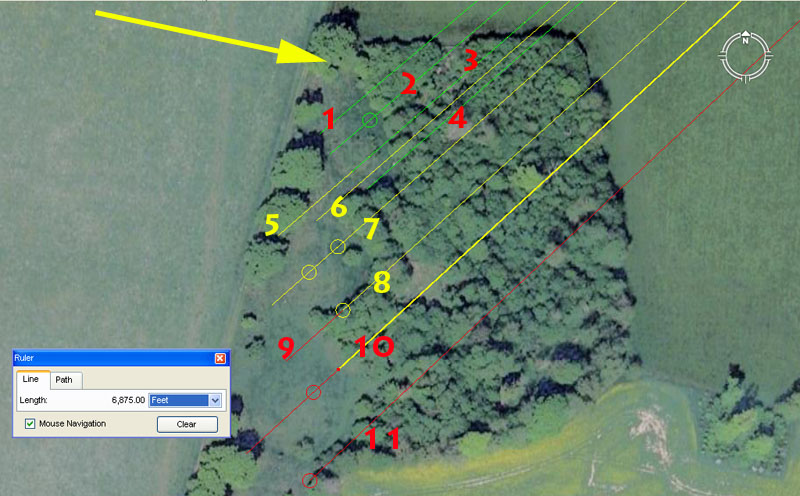Northeast of Coneybury Henge are the very impressive King Barrows lying on an approximate North-South line. In the above picture only three of them are shown. At the top of the picture is an arrow pointing to the apparent edge of the northernmost mound. The mound itself appears to be quite elongated and if this position represents its northern tip, then the spot sits 6600' (1.25-miles) from Durrington Walls centre at an angle of 230.4-degrees from the henge. This is a dynamic navigational code related to the equatorial circumference of the Earth. The return angle (50.4-degrees) is a very versatile navigational and lunar code simultaneously. The other tutorials built into barrow 1 (green lines) would have been:

1. The distance is 6666.666666' and the return angle back to Durrington Walls is 49.7664-degrees.

The distance is 1/3rd of 20,000'.

The angle code relates to the 24883.2-mile equatorial circumference of the Earth, of which 4976.64-miles would represent 1/5th part or 72-degrees of arc.

2. The distance is 6600' and the angle is 49.5-degrees.

The distance is 1000 chains, 10 furlongs / furrowlongs or 1.25-miles.

The angle code relates to the 24750-mile equatorial circumference, of which the sum of 4950-miles would be 1/5th part.

2b. The distance is 6666.6666' and the angle remains 49.5-degrees.

This distance onto the mound is 1/3rd of 20,000'.

3. The length is 6666.6666' and the angle from Durrington Walls to this spot is 229.166666-degrees.

The angle code (2291/6th) relates to the equatorial circumference of the Earth under the 24750-mile assignment and 22.9166666-miles (2211/12ths) would be 1/3rd of 1-degree of equatorial arc. This distance equates to 121000' and represented a nicely rounded value for navigators to refer to. By consequence, the value was only 40' longer than the 120960' distance (18 Irish miles of 6720' each) that represented 1/3rd of 1-degree of arc for a world configured (for navigational purposes) to be 24883.2 Greek miles in equatorial circumference. Under the Irish mile reading a degree of arc was a perfect 54 Irish miles.

4. The distance is 6666.6666' and the angle is 48.8888-degrees.

The angle is simply a 1/9th expression of 440.

Mound 2 (yellow lines).

5. The distance is 6804' and the angle is 229.16666-degrees.

This length is in homage to the 6804-day lunar nutation cycle. It would also equate to 1/19200th of the equatorial circumference of the Earth under the 24883.2-Greek mile assignment.

A position slightly North on this mound was probably marking 49.21875-degrees (497/32nds) and relate to fraction values of the lunar year

6. The distance is 6750' and the angle is 2291/11th-degrees.

The value 6750 is part of a lunar and navigational progression simultaneously.

The angle provides an expression related to 1-degree of arc in a world configured to 24883.2 Greek miles, wherein 22 10/11ths miles of 5280' equates to 1/3rd of 1-degree of arc.

7. The distance remains 6750' and the angle is 48.6-degrees.

The degree angle is provides a mathematical progression that is both navigational and lunar coding simultaneously.

7b. The distance is 6804' and the angle remains 48.6-degrees.

The length code is in homage to the lunar nutation cycle of 6804-days.

7c. The distance is 6875' and the angle remains 48.6-degrees.

The length is in homage to 1-degree of arc (68.75-miles) for a world configured to be 24750-miles in equatorial circumference.

8. The length is 6804' and the angle is 48-degrees.

The angle is self explanatory.

Mound 3 (red lines).

9. The length is 6912' and the angle remains 48-degrees.

In a world configured to be 12 X 12 X 12 X 12 X 1.2 miles in circumference, 1-degree of arc is 69.12-miles.

10. The length is 6875' and the angle is 47.52-degrees.

The sum of 47520' would be 9-miles of 5280' each.

10b. The distance is 6912' and the angle remains 47.52-degrees.

10c. The distance is 7040' and the angle remains 47.52-degrees.

The distance is 11/3rd miles of 5280'.

11. The distance is 7000' and the angle is 226.8-degrees out from Durrington Walls.

The angle coding is both lunar and navigational simultaneously and 3 side values of the Great Pyramid @ 756' per side = 2268'.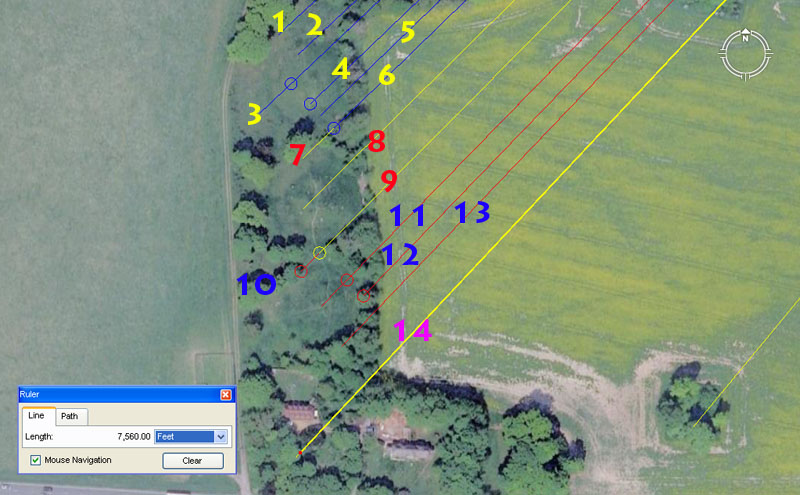The four more southerly mounds of the King Barrow complex are shown above. The apparent codes are:

Fourth mound

1. The distance is 7087.5' and the angle is 47.25-degrees.

The Khafre Pyramid of Egypt has a side length of 708.75', the half value of which is 354.375'. There are 354.375-days in a lunar year.

The Khafre Pyramid of Egypt is 15/16ths the length of the Great Pyramid. Therefore 756' ÷ 16 = 47.25' ... X 15 = 708.75'. The design height of the Khafre Pyramid (which was built to be two 3,4,5 triangles back-to-back) was 472.5'.

2. The length is 7087.5' and the angle is 46.875-degrees.

The angle (like so many others) is dual coded and provides dynamic mathematical progressions @ 46.875-degrees or 180-degrees opposed at 226.875-degrees.

(a) @ 46.875-degrees the value is both lunar and navigational simultaneously.

(b) @ 226.875-degrees the value relates to navigation under the "11" series of numbers, based upon an equatorial circumference of 24750-miles. For example, the sum of 22687.5' would be 1/16th of 1-degree of arc.

3. The distance is 7128' and the angle is 46.656-degrees.

A distance of 71280' would equate to 12 Scottish miles of 5940' or 13.5 English miles of 5280'.

From the centre of Stonehenge out to a marker on the Avenue is 233.28' or the radius of a circle 466.56' in diameter. The diameter of such a circle would be 270 Egyptian Royal Cubits of 20.736" each or 135 for the radius measurement.

The post marker sets up a series of PHI reducing, concentric circles that define the diameters of the main circles within Stonehenge. Therefore 466' (rounded) ÷ 1.6180339 = 288 (the Aubrey Circle diameter) ÷ 1.6180339 = 178.2' (the diameter of "Y" Holes) ÷ 1.6180339 = 110 (the diameter of the Sarsen Circle).

The 46656 value was of such immense importance that it was incorporated into the design angle that existed between Stonehenge and its distant out benchmark on the crown of Barbury Hill, over 20-miles northwards. The azimuth angle is 4.6656-degrees.

Under the Sumerian-Babylonian weight standard for dry goods (including gold), the single talent was 466560 grains and the double standard was 933120 grains. Under the navigational system that configured the world to be 12 X 12 X 12 X 12 X 1.2 Greek miles (130636800') the sum of 46656' (9.6 Roman miles) would equate to 1/2800th part.

 SUMERIAN-BABYLONIAN ROYAL DOUBLE STANDARD. 1 Talent………933120 grains, equals: 60 Mina…….. @ 15552 grains, or: 3600 Shekel… @ 259.2 grains. SUMERIAN-BABYLONIAN ROYAL SINGLE STANDARD. 1 Talent……… 466560 grains, equals: 60 Mina……… @ 7776 grains, or: 3600 Shekel….. @ 129.6 grains.

3b.The distance is 7200' and the angle is 46.656-degrees.

The distance is half a geomancer's mile (14400').

4. The distance is 7128' (1.35 miles) and the angle is 46.28571430-degrees (462/7ths).

The degree angle relates to navigation and Precession of the Equinoxes codes and rounds to a full number at each seventh stage of a mathematical progression.

5. The distance is 7128' and the angle is 46.08-degrees.

The degree angle is navigational coding and 46.08-miles would equate to 1/540th of the 24883.2-mile circumference of the Earth.

6.The distance is 7128' and the angle is 45.833333-degrees.

The angle code is related to the ancient league of 16500', of which the sum of 45.8333333 (550") would equate to 1/360th part. This mathematical principle was obviously exploited in navigation and positional plotting at sea. A Greek mile of 5250' of linear travel (diameter or leg) creates a circle of 16500' using PI @ 22/7ths and one degree of arc on that circumference is 45.83333333' (455/6ths). This type of calculation would have been done on the plotting chart, at the completion of each leg, to stay abreast of the degree angle back to the point of departure or onwards to the destination.

Fifth mound.

7. The distance is 7200 and the angle is 45.833333-degrees.

Both distance and angle explained,.

8. The distance is 7257.6' and the angle is 45.36-degrees.

In a world of 24883.2 Greek miles, the sum of 72576' would be 1/1800th part or 1/5th of 1-degree of equatorial arc.

The vertical height of the Great Pyramid to the top of it's flat floor altar was 453.6' (navigational code). The length of the Great Pyramid @ 756' equates to 9072" or 4536" X 2.

9. The distance is 7290' and the angle is 44.8-degrees.

This distance is 1.5 Roman miles, 1500 Roman Paces or 7500 Roman feet.

The angle is navigational and 4480' would equate to 1/81st part of 1-degree of equatorial arc under the 24883.2 Greek mile system. The 112, 224, 448 progression was used in weights standards from early times and 112 lbs (pounds) became our cwt (hundredweight) and 2240 lbs became our ton.

Sixth Mound.

10. The distance is 7333.33333' and the angle is 44.8-degrees.

The distance is an expression of the ancient English league and 2.25 X 7333.3333' = 16500'.

11. The distance is 7290' and the angle is 44.296875-degrees (4419/64ths).

The distance is 1.5 Roman miles. The 729 value is very useful in lunar cycle calculations and the Bush Barrow Lozenge artefact, found at Normanton Downs, is 7.29" long (1/8th of a Roman Pace of 58.32"). It's also useful to note that the Druidic Calendar of Coligny of France is 58.32" in length.

The sum of 44.296875-days would be 1/8th of a lunar year of 354.375-days.

11b. The distance is 7382.8125 and the angle is 44.296875-degrees (4419/64ths).

The distance code or tutorial relates to the lunar month and year and 7382.8125 (738213/16ths) is 1/48th of 354375.

12. The distance is, again, 7290' and the angle is 44-degrees.

The value 44 is a part of the "11" progression of numbers and was very valuable to navigation. The sum of 440' would equate to 1/12th of an English mile.

13. The distance is 7382.8125' and the angle is 43.75-degrees.

The number generated in this degree angle was very important to navigation and calculations of lunar cycles. The sum of 437.5' would equate to 1/12th of a Greek mile. The Romans had two forms of the foot including 11.664" (for lunar and volume calculations) and 11.66666" (for overland distances). The sum of 43750' equates to 9 Roman overland miles.

Seventh Mound.

14. The distance is 7560' and the angle is 43.2-degrees.

The Great Pyramid is 756' in length and this constitutes 1/8th of 1-minute of equatorial arc under the "6&7" navigational system. This distance out from Durrington Walls centre equates to 1.25-minutes of equatorial arc or 75-seconds. This distance is 1/17280th of the equatorial circumference under that navigational system. Remember: a cubic foot is 12" X 12" X 12" = 1728. The largest Egyptian Royal cubit found by Petrie was 1.728' or 20.376".

The angle code relates to very much the same thing and the 756' length of the Great Pyramid also equates to 432 Hebrew or Celtic Royal cubits of 21".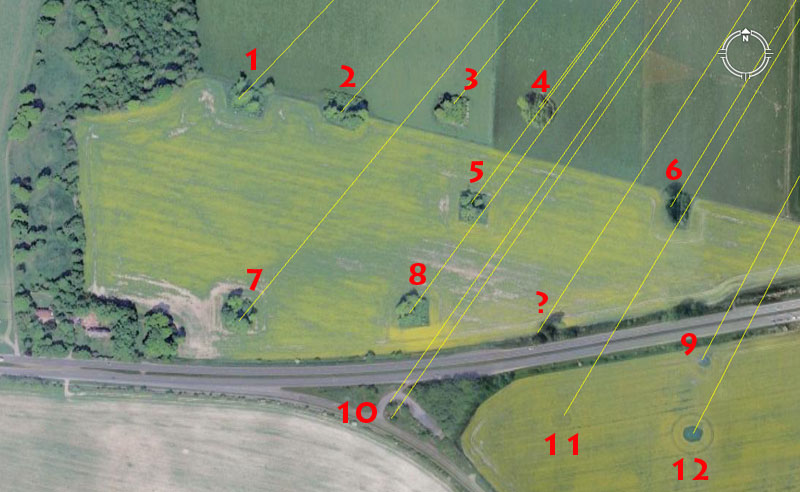To the immediate East of the large King Barrows are many smaller surveying-related marker mounds, which were very carefully positioned to sit at exact distances and angles from the centre of Durrington Walls Henge. Some shown in the bottom right of the picture are reduced to little more than crop-marks now, thousands of years after they were first erected for the "open-air university", however, they can still divulge their original codes of position with absolute clarity. Here are the centre-codes for this dynamic grouping of surveying markers:

1. The distance is 6534' and the angle is 43.75-degrees.

The 6534 value is one of the foremost in importance to the ancient parcel of special numbers and relates to the equatorial circumference of the world under the 24750-mile assignment. This distance at Durington would be 1/20000th of the circumference under that navigational method.

2. The distance is 6336' and the angle is 41.25-degrees.

The value 6336 was a conversion number for identifying the equatorial circumference of the world in "feet" under 3 separate navigational systems. To use this value as a mnemonic device, one considers the 6336 value to be in Egyptian Royal cubits, of which there were 3 types.

1. 6336 X 20.61818182" (this is the shortest of the 3 and an example of this is found in the Turin Museum) = 130636.8. Under the Great Pyramid's literal assignment the world was 130636800' around.

2. 6336 X 20.625" = 130680. Under the Great Pyramid's second assignment (by extending its length symbolically by 3") the world could be read as 24750-miles or 130680000'. The existence of the 20.625 inch Royal Cubit is confirmed by the careful measurements of Sir William Flinders Petrie in the King's Chamber of the Great Pyramid. Petrie concluded that the room was built according to a cubit of 20.620 inches, plus or minus .005 of an inch. The upper scale of his estimate is, therefore, 20.625 inches (see The Pyramids and Temples of Gizeh, by Sir William Flinders Petrie).

3. 6336 X 20.736 = 131383.296. Under the system where the Earth is viewed as being 12 X 12 X 12 X 12 X 1.2 miles of 5280' each, the total equates to 131383296'. The existence of the 20.736 inch cubit is confirmed by the paving slab widths at the base of the Great Pyramid, which comply to 20 X 1.728 feet. This increment of length was positively identified by Sir William Flinders Petrie to also be present in the length of the coffer found in the Khafre Pyramid of Egypt. The coffer measured 103.68-inches or 5 cubits of 20.736-inches each.

The distance of 6336' equates to 96 chains or 9.6 furlongs / furrowlongs.

3. The distance is 6075' and the angle is 218.75-degrees, extending outwards from Durrington.

This distance out from Durrington Walls centre is strong lunar and navigational coding simultaneously. This length equates to 1.25 Roman miles or 1250 Roman Paces of 58.32" each. There would be 112 intervals of 60.75-days in the 6804-day lunar nutation cycle, 42 intervals of 60,75-days in the 2551.5-day (7.2 lunar years) period monitored within the lunisolar Sabbatical calendar alongside 2556.75-days (7 solar years), or 5.83333 intervals of 60.75-days in a lunar year. Remember, the Roman overland Pace was 58.3333" ... and of course the whole sophisticated interplay of special numbers was coded into the English landscape thousands of years before the epoch of the Romans.

The angle of 218.75 is part of the same mathematical progression as that of 4375, producing lunar and navigational numbers.

4. The distance is 5906.25' and the angle is 36.525-degrees.

The distance coding is lunar and the sum or 59.0625-days would equate to two lunar months. Under the 3,4,5, triangle method by which the Khafre Pyramid of Egypt was built, the diagonal slope length (hypotenuse) was 590.625'.

The degree angle is in homage to the solar year at 365.25-days.

5. The distance is 6300' and the angle is 36.45-degrees.

This distance is 10 Greek stadiums (stadia) of 630' each. The circumference of the inner rim of the Sarsen Circle lintels at Stonehenge was designed to convey 315' or half a stadia. This distance out from Durrington Walls equals 1.2 Greek miles of 5250' each. The same distance occurs centre to centre between the Octagon of Newark, Ohio and adjacent earthmound structures. This length would be 1/20736th of the equatorial circumference under the Great Pyramid's literal assignment. Remember, the longest Egyptian Royal cubit was 20.736".

The 36.45 value identified in the degree angle is lunar and navigational coding and part of the same mathematical progression that produced 218.75 and 437.5, recently discussed.

6. The distance is 5940' and the angle is 211.2-degrees out from Durrington Walls.

This distance is 1 ancient Scottish mile or 1.125 English miles. It is also 9 furlongs or furrowlongs.

The angle code is part of the same mathematical progression that produced 6336 (discussed). The sum of 2112' would equate to 32 chains or 3.2 furlongs / furrowlongs.

7. The distance is 7087.5' and the angle is 39.6-degrees.

This coding is some of the most dynamic on the landscape and the 7087.5' distance is exactly the same as the distance at from the central grand obelisk position at Avebury Henge to the doorway line of obelisks at East Kennet longbarrow. The Khafre Pyramid of Egypt had a design length of 708.75' (15/16ths the length of the Great Pyramid @ 756'). The sum of 708.75-days would equate to two lunar years.

The degree angle value is part of a mathematical progression that relates to the radius and diameter of the Earth, which is 7920-miles (radius 3960-miles).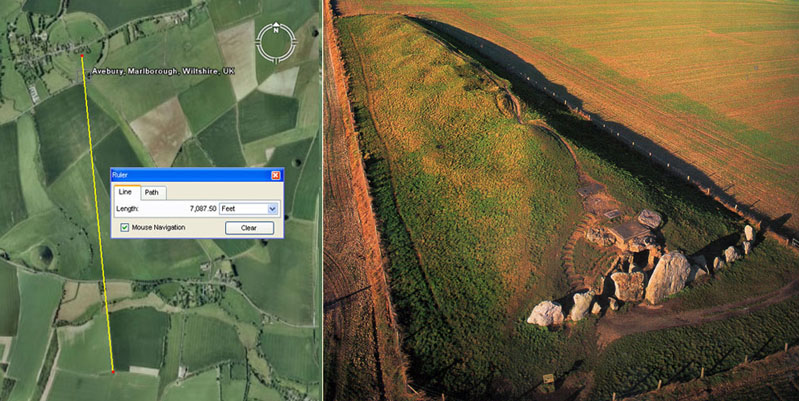Left: From the obelisk at Avebury Henge a line runs southwards and touches down on the line of obelisk boulders adjacent to the doorway into West Kennet Longbarrow. The intended or coded distance for this length was very definitely 7087.5' (10 X the design length of Khafre Pyramid). The distance is fully verified by the return angle back to the obelisk at Avebury Henge, which is 354.375-degrees (354.375 - 3543/8-days = the lunar year and this value in feet is half the length of Khafre Pyramid).

Right: The row or wall of stones in front of the doorway into West Kennet Long Barrow runs towards Avebury Henge. The giant obelisk of the henge sat 7087.5' away at an azimuth angle of 354.375-degrees from the stone row by the doorway. Both in distance and angle the coding is lunar. Alternatively, the centre of the prominent "V" dip further along the barrow's ridge path sat at an azimuth angle of 175° from the henge's obelisk (a very dynamic, ancient navigational and lunar value). From the "V" dip at West Kennet Longbarrow, due North was the eastern edge of Avebury Henge's embankment. Photo by Jason Hawkes, Prehistoric Britain from the Air, by Janet & Colin Bord, 1997.

8. The distance is 6720' and the angle is 36-degrees.

This distance is one ancient Irish mile and 54 or these would be 1-degree of arc for a world configured as 24883.2 Greek miles.

The angle is 1/10th of 360-degrees.

? It is uncertain if this position beside the highway is a dissected, former mound. It seems to comply to a prominent ancient code @ 6534' and an angle of 212.625-degrees out from Durrington Walls.

The degree angle is lunar and relates to the lunar nutation cycle of 6804-days, of which 212.625-days equals 1/32nd part. As with navigational calculations, cycles could be monitored and followed on a daily basis using a calibrated disc of both 360 & 320 positions. Knowing the exact position of the Moon within the long cycle was very important to ancient mariners, both for celestial navigation and as an indicator of what the weather might do.

9. The distance is 6336' and the angle is 28-degrees.

The distance coding has been explained.

The angle value forms part of a mathematical progression much used in navigation or Sabbatical calendar calculations.

10. The distance is 7000' and the angle is 35-degrees.

Both distance and angle are related and a part of the same mathematical progression, with 35 being half of 70. Again, the numbers generated in a progression related directly to lunisolar, Sabbatical calendar calculations.

10b. The distance is 7040' and the angle is 34.71428571 (345/7ths).

The distance is 11/3rd miles.

The angle provides a "7" based mathematical progression, which resolves to a lunar or navigational value with each seventh stage. The sum of 345/7ths feet would equate to 1/140th of a Roman mile. There would be 196 occupancies of 345/7ths days in the 6804-day lunar nutation cycle.

11. The distance is 6720 and the angle is 30.72-degrees.

The sum of 307.2-miles would be 1/81nd of the 24883.2-mile equatorial circumference.

12. The distance is 6562.5' and the angle is 27.216-degrees.

This distance provides a value that is both lunar and navigational simultaneously and 656.25' would be 1/8th of a Greek mile or 6.5625-days (69/16ths) would be 1/54th of a lunar year.

The degree angle is navigational and the sum of 27216' would be 1/4800th of the 24883.2 Greek mile circumference.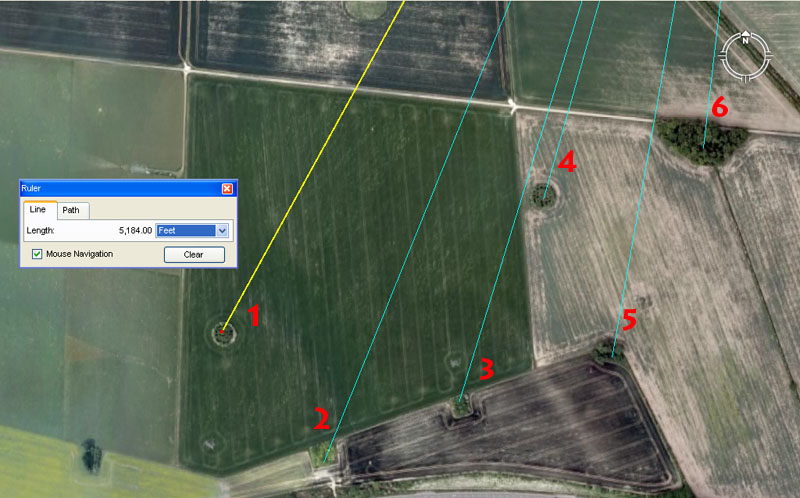Yet further ENE are these field markers which, again, are set very precisely out from the centre of Durrington Walls Henge to contain distance and angle codes used in navigation and cyclic astronomy. Here is what was encoded into these positions:

1. The distance is 5184' and the angle is 28.8-degrees.

The slope angle on the Great Pyramid of Egypt is 51.84-degrees . The Great Pyramid @ 756' long is 72 Hebrew reeds in length. It therefore covers an area of 72 X 72 reeds or 5184. Alternatively, the sum of 51.84-years is 1/500th of the 25920-year duration of the Precession of the Equinoxes.The azimuth angle that the Octagon of Newark Ohio, USA (a very sophisticated and large geometric earthmound complex) lies on is 51.84-degrees.When navigating at sea using the ancient British league of 16500' (3.125-miles of 5280') for linear legs of distance covered, the plotting circle generated per league was 51840'. This plotting circle was then fully divisible by 360° and as long as legs of straight line travel were done in accumulations of full leagues or lesser divisions thereof before a heading change, then accurate degree angle calculations related to positions at sea were easily facilitated. By this mathematical method the navigator could always keep track of the angle back to the point of departure or onwards to the destination.

With regards to the 28.8 value arising in the degree angle:

Herodotus, the Greek historian who visited Egypt circa 454 BC. has been severely criticised by pyramidologists/ Egyptologists for his several "uninformed/ misinformed" statements relating to the pyramids. He said, amongst other things, that 'the surface area of each face was equal to the square of the height'. He also said that 'the surface area of each face was 8-Egyptian acres'. For making these observations, Herodotus has been severely derided by pyramidologists, who can't make his statements work...but as it turns out he was totally correct.

* The length along the base of the Great Pyramid is 756 feet; the diagonal length up a face side runs for 576 feet to a flat floor at the top which is, or once was, 44 feet long (a bit of a jumbled mess now). This configuration gives a surface area, per face, of 230400 square feet, which means an ancient Egyptian Pyramid Acre (according to Herodotus) was 28800 square feet.

* Although the Great Pyramid has a flat floor on top (and always has) it can still be symbolically read as having a height complying to the point where lines running up each diagonal face would intersect. This position would be 27 feet in the air above the present flat floor altar atop the pyramid. This is what Herodotus was talking about in the design geometry. Therefore, with 230400 square feet representing the square footage value of each face, the square root of this should be the height. Inasmuch as 480 X 480 = 230400, the design height (if it was a complete pyramid with an apex) would be 480 feet of vertical height...which is true (to a tolerance of about 8 inches shortfall...it's closer to 481 feet in trigonometric calculation, based upon a true face angle of 51.84-degrees).

From the earliest times, long before the Nubian invasions, Egypt (and its environs) was fully a Caucasoid nation. As it got more & more arid there was a huge exodus out of Egypt, with many of its people emigrating to cousin nations in Continental Europe & Britain. The mathematical principles and standards remain the same, from the Eastern base of the Mediterranean to Britain. Whether it be the pyramids of Egypt or great henge & mound complexes of Britain, the code-bearing measurements and angles remain the same.

2. The distance is 5443.2' and the angle is 22-degrees.

The total of 54432' would equate to 1/2400th of the 24883.2 Greek mile equatorial circumference.

The degree angle code relates to an 11 progression and 22-English miles would equate to 1/1125th of the 24750-mile equatorial circumference. It will be recalled that 11.25-degrees is 1/32nd of a 360-degree circle.

2b. The distance is 5500' and the angle is 22-degrees.

The distance is 1/3rd of an ancient English league of 16500' (3.125-miles). The sum of 550-miles would be 1/45th of the 24750-mile equatorial circumference. It will be recalled that 45-degrees is 1/8th of a 360-degree circle.

3. The distance is 5062.5' and the angle is 17.01-degrees.

The distance is lunar coding and 50.625-days (505/8ths) would be 1/7th of the 354.375-day lunar year.

The angle is also lunar coding and 1701-days would equate to 1/4th of the 6804-day lunar nutation cycle. Alternatively, the sum of 1701-days would equate to 4.8 lunar years. The southern circle of Avebury Henge was anciently designed to represent a diameter of 340.2' (170.1 X 2) ... and the same diameter code was built into the Ring of Brodgar in Scotland.

4. The distance is 4125' and the angle is 15.75-degrees.

The distance is navigational and the sum of 4125' equates to 1/4th of an ancient English league.

The angle is also navigational and 1575' equated to 1/3rd of a Greek mile. The sum of 157.5' was 1/2 a Greek stadia. In a 360-degree circle, 157.5-degrees would be SSE.

0 (N), 22.5 (NNE), 45 (NE), 67.5 (ENE), 90 (E), 112.5 (ESE), 135 (SE), 157.5 (SSE), 180 (S), 202.5 (SSW), 225 (SW), 247.5 (WSW), 270 (W), 292.5 (WNW), 315 (NW), 337.5 (NNW), 360 (N).

5. The distance is 4725' and the angle is 10.08-degrees.

The increment of 1/16th of the Great Pyramid (756') is 47.25'. The increment of 1/15th of the Khafre Pyramid (708.75') is 47.25'. The design height of the Khafre Pyramid was 472.5'.

The angle coding is navigational and 100.8' is 1-second of equatorial arc under the Great Pyramid's literal geodetic system. One of the internal cross-measures, lintel to lintel of the Sarsen Circle at Stonehenge is 100.8'.

6. The distance is 3780' and the angle is 186.624-degrees extending out from Durrington Walls Henge.

The distance is navigational and 1/2 of the length of the Great Pyramid is 378'. The diameter that best describes the Stonehenge site's overall diameter from NE to SW is 378'.

The angle code is navigational and the sum of 186624' would equate to 1/700th of the 24883.2 Greek mile equatorial circumference.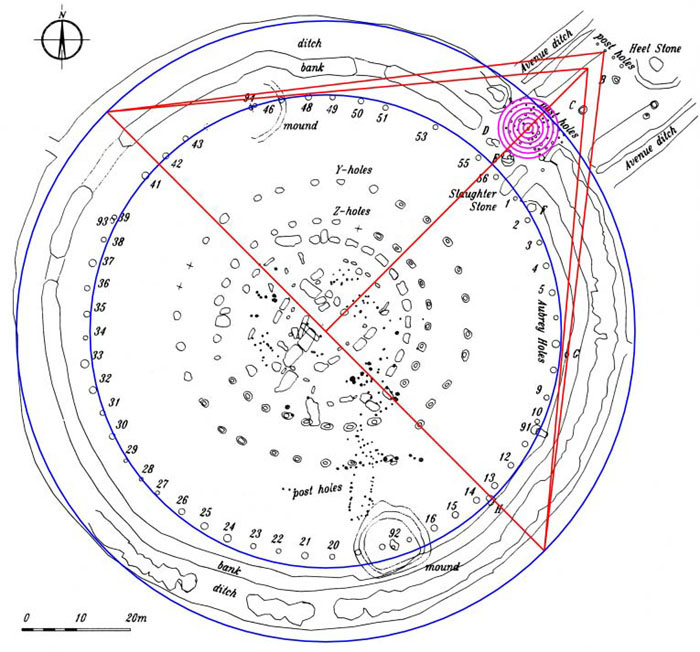The outermost circle shown has a diameter of 378 feet and this value is 1/2 the base length of the Great Pyramid. Note how the circle encases Stonehenge's circles from 45-degrees through 225-degrees. Prof. Alexander Thom commented on how British stone circles were built to be slightly elliptical and the diameter of the Stonehenge site complies to 384' from 135-degrees azimuth through 315-degrees. This was done to achieve 2 dynamic diameter codes 90-degrees opposed.

When an exact 1/2 scale geometric representation of the Great Pyramid is incorporated into the Stonehenge monument's dimensions in this way, a number of excellent relationships are seen to occur. Lines extending from both sides of the baseline and set to an angle of 51.84-degrees (the Great Pyramid's side diagonal angle) converge upon and precisely touch a post marker on the Avenue. The post was obviously placed to record this relationship to the Great Pyramid. Alternatively, lines from the base, which brush the edge of the Aubrey Circle, converge upon a point mathematically consistent with the Pyramid's flat floor top. It is quite obvious that the Stonehenge design layout incorporated geometric features directly associated with the Great Pyramid. This is a perfect half scale, two dimensional replica of the Great Pyramid, supposedly placed there in the English landscape (according to the peer-reviewed wisdom of our establishment archaeologists) about 400-years before the Great Pyramid was built.

In reality, the Great Pyramid is far, far older than our archaeologists and historians are allowed to admit.

Continue